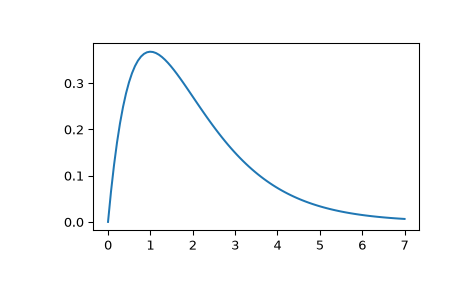# scipy.signal.impulse¶

scipy.signal.impulse(system, X0=None, T=None, N=None)[source]

Impulse response of continuous-time system.

Parameters
systeman instance of the LTI class or a tuple of array_like

describing the system. The following gives the number of elements in the tuple and the interpretation:

• 1 (instance of `lti`)

• 2 (num, den)

• 3 (zeros, poles, gain)

• 4 (A, B, C, D)

X0array_like, optional

Initial state-vector. Defaults to zero.

Tarray_like, optional

Time points. Computed if not given.

Nint, optional

The number of time points to compute (if T is not given).

Returns
Tndarray

A 1-D array of time points.

youtndarray

A 1-D array containing the impulse response of the system (except for singularities at zero).

Notes

If (num, den) is passed in for `system`, coefficients for both the numerator and denominator should be specified in descending exponent order (e.g. `s^2 + 3s + 5` would be represented as `[1, 3, 5]`).

Examples

Compute the impulse response of a second order system with a repeated root: `x''(t) + 2*x'(t) + x(t) = u(t)`

```>>> from scipy import signal
>>> system = ([1.0], [1.0, 2.0, 1.0])
>>> t, y = signal.impulse(system)
>>> import matplotlib.pyplot as plt
>>> plt.plot(t, y)
```